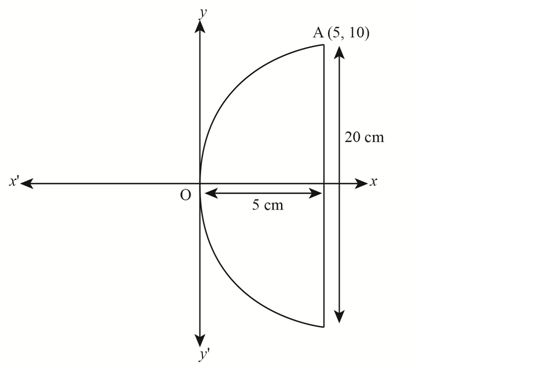If a parabolic reflector is 20 cm in diameter and 5 cm deep, find the focus.

Asked by Pragya Singh | 1 year ago |  126

##### Solution :-

We know that the origin of the coordinate plane is taken at the vertex of the parabolic reflector, where the axis of the reflector is along the positive x – axis.

Diagrammatic representation is as followsWe know that the equation of the parabola is of the form y2 = 4ax (as it is opening to the right)

Since, the parabola passes through point A(10, 5),

y2 = 4ax

102 = 4a(5)

100 = 20a

a = $$\dfrac{100}{20}$$

= 5

The focus of the parabola is (a, 0) = (5, 0), which is the mid – point of the diameter.

Hence, the focus of the reflector is at the mid-point of the diameter.

Answered by Abhisek | 1 year ago

### Related Questions

#### An equilateral triangle is inscribed in the parabola y2 = 4ax,

An equilateral triangle is inscribed in the parabola y2 = 4ax, where one vertex is at the vertex of the parabola. Find the length of the side of the triangle.

#### A man running a racecourse notes that the sum of the distances from the two flag posts from him is always

A man running a racecourse notes that the sum of the distances from the two flag posts from him is always 10 m and the distance between the flag posts is 8 m. Find the equation of the posts traced by the man.

#### Find the area of the triangle formed by the lines joining the vertex of the parabola x2 = 12y to the ends

Find the area of the triangle formed by the lines joining the vertex of the parabola x2 = 12y to the ends of its latus rectum.